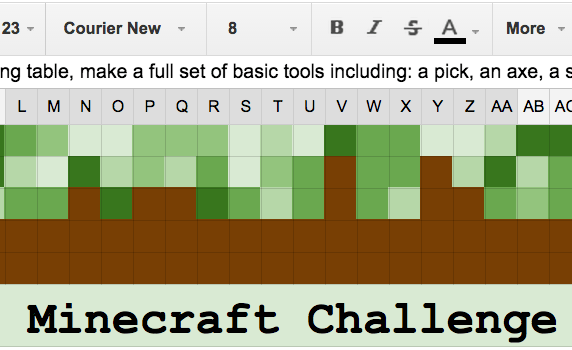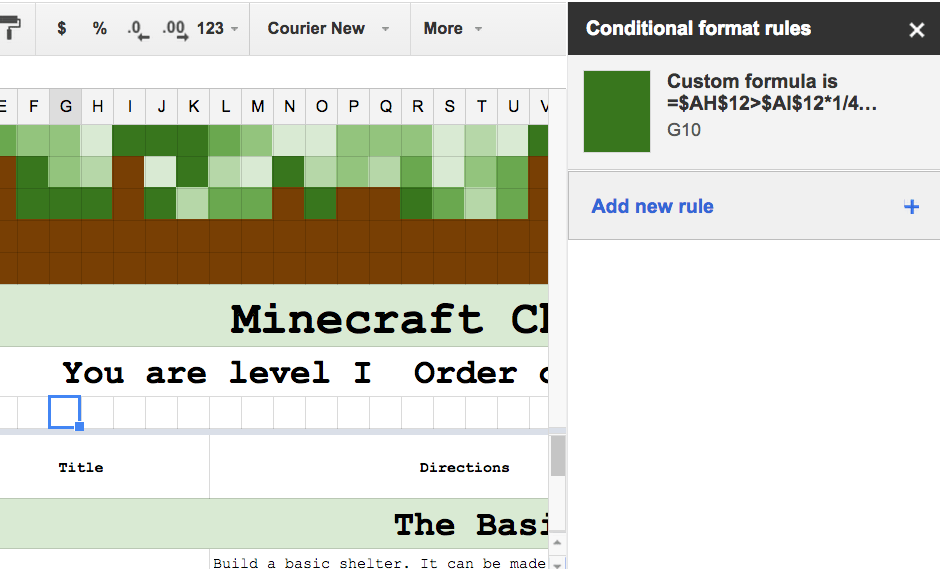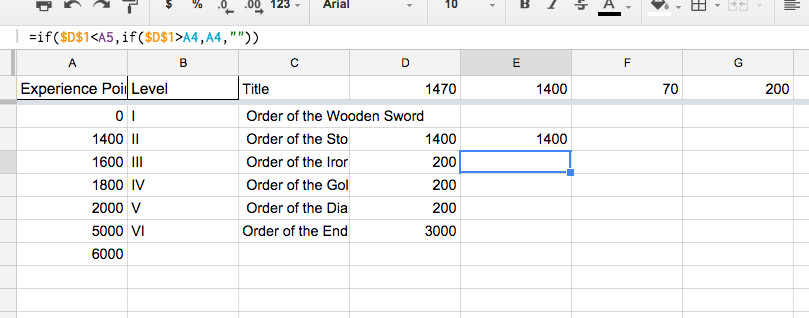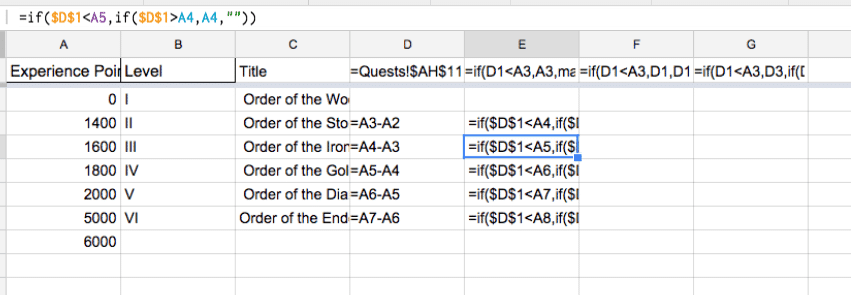First let me thank Lucas Gillespie for creating the Minecraft Challenge and sharing it under a Creative Commons license. If you’re not familiar with Lucas’ work in Game Based Learning I highly encourage you to check out his website: http://edurealms.com/

Lucas shared the Minecraft Challenge with me as a Google Doc. It is a list of quests for things to do in Minecraft. Any sort of table or list screams to be in a spreadsheet so with his permission I recreated the challenge in Google Sheets.

# Progress Bar

While creating the spreadsheet I noticed that the challenge required 1400 points to level up to level 2. With quests being worth 20, 50 or 100 points it was going to take awhile to reach the next level. I thought that seeing some progress would make the challenge more fun.

For the spreadsheet I used conditional formatting to create a progress bar so the students would see how far they were from reaching the next level. As students mark off their quest completion a formula calculates what percentage of the way they are to the next level. Conditional formatting checks if the value exceeds a certain percentage and if it does colors the cell green.

After the student levels up the progress bar goes back to the beginning. In this particular challenge students need 1400 points to get to level 2. However they only need 200 additional points to reach each subsequent level. Once the student reaches level 2 the progress bar increments significantly faster.

# Creating a Progress Bar

## Resize Columns

For this particular spreadsheet I resized the cells to small squares. This not only let me create the Minecraft design at the top of the spreadsheet but created a nice row of squares to create a progress bar. Resizing the columns would be recommended if you’re making a progress bar.

## Create a Table

Somewhere you need to sum up the points the student earns when they check off values. I hid a column where I wrote an equation to show points if a student marked off a quest. =if(AE14=””,””,AB14) This says if the mark off column is blank do nothing. Otherwise, take the value of the XP for that quest. I then sum the points in that column and hide the column.

In a cell somewhere in the spreadsheet write a formula to show what percentage of the way the student is to the next level.

(Number of points earned) – (Previous level maximum) = (Points toward next level)

## Incremental

The banner I created is 42 cells wide. I did not plan that number, it just happened to be the width of my visible window. This means each cell is 1/42 of the distance to the next level.

For EACH cell you need to set the conditional formatting.

## White Font

In sequentially numbered each of the 42 cells and used a white font. This makes it so you can not see number in the cell. Each number represents the fraction of the distance to the end of the progress bar. For example the 14th cell is 14/42 of the way to the end.

## Conditional Formatting

Right clicking on the first cell in the progress bar reveals an option for conditional formatting. A side panel will open to add a new rule.From the side panel choose “Custom formula is” from the drop down list under “Format cells if…” The cell will be highlighted green (with matching font color so the number in the cell stays hidden). Starting with an equals sign the conditional equation says that if the number of incremental points the student has earned is greater than the number of points between the levels, multiplied by 1/42 to represent each square in the progress bar and multiplied by the value in the cell for that spot on the progress bar then to color the cell green.## Fun with Math

It did take me a few iterations to get the formula correct so that the cells turned green as the student leveled up. The really tricky part was figuring out how to get it to recognize that the student was at the next level and to change the value the number was being compared against. There are many ways to accomplish this but what I did was create a column next to the table of values and incremental values. I wrote an if statement that if the students total points was less than that levels value but greater than the previous levels maximum to display the previous levels maximum. This allowed me to subtract the previous levels maximum points from the total points the student had earned. This tab is hidden in the Minecraft Quest sample. Go to the “View” menu to reveal the hidden sheet.Also under the “View” menu is the option to turn on “All formulas.” This will allow you to see what formulas I used. There are definitely other ways to accomplish this.## Subscribe to the Alice Keeler Newsletter